Trial ends in

# Finite Element Modeling for the Simulation of the Quasi-Static Compression of Corrugated Tapered Tubes

Published: January 6, 2023 doi: 10.3791/64708

### Abstract

In this study, the quasi-static compression performance of tapered tubes was investigated using finite element simulations. Previous studies have shown that a thickness gradient can reduce the initial peak force and that lateral corrugation can increase the energy absorption performance. Therefore, two kinds of lateral corrugated tapered tubes with variable thicknesses were designed, and their deformation patterns, load displacement curves, and energy absorption performance were analyzed. The results showed that when the thickness variation factor (k) was 0.9, 1.2, and 1.5, the deformation mode of the single corrugated tapered tube (ST) changed from transverse expansion and contraction to axial progressive folding. In addition, the thickness gradient design improved the energy absorption performance of the ST. The energy absorption (EA) and specific energy absorption (SEA) of the model with k = 1.5 increased by 53.6% and 52.4%, respectively, compared with the ST model with k = 0. The EA and SEA of the double corrugated tapered tube (DT) increased by 373% and 95.7%, respectively, compared with the conical tube. The increase in the k value resulted in a significant decrease in the peak crushing force of the tubes and an increase in the crushing force efficiency.

### Introduction

Crashworthiness is an essential issue for lightweight automobiles, and thin-walled structures are widely used to improve crashworthiness. Typical thin-walled structures, such as round tubes, have good energy absorption capacity but usually have large peak forces and load fluctuations during the crushing process. This problem can be solved by introducing axial corrugations1,2,3. The presence of corrugations allows the tube to plastically deform and fold according to a predesigned corrugation pattern, which can reduce the peak force and load fluctuations4,5. However, this stable and controlled deformation pattern has a drawback: the energy absorption performance decreases. To improve the energy absorption of axial corrugated tubes, researchers have tried many methods, such as using a functional gradient design in the wavelength6,7 and amplitude8, using filling foam9,10, forming multichamber and multiwall structures11, and forming combined tubes12.

In addition, researchers have designed lateral corrugated tubes by introducing corrugations into the cross-section of circular tubes13,14,15,16. The existence of lateral corrugations greatly improves the energy absorption performance of the tube17,18,19. Eyvazian et al.20 compared the crashworthiness of lateral corrugated tubes and ordinary circular tubes and showed that lateral corrugated tubes had a better energy absorption capacity. One reason for this observation is that the lateral corrugation strengthens the tube wall, which makes it more resistant to plastic folding. In addition, the corrugated wall of the plastic folding part flattens, and this flattening also absorbs energy. However, the high initial peak force is a disadvantage of this type of tube, and this high initial force may seriously affect the safety of the passengers being transported.

Functionally graded structures have a natural advantage in reducing the peak force. Common functionally graded thin-walled tubes are usually formed by changing the geometric parameters (e.g., the diameter and wall thickness)21. The most prevalent structures for which the diameter is changed are tapered tubes, including circular tapered tubes22, square tapered tubes23,24,25, polygonal tapered tubes26,27, axial corrugated tapered tubes28,29,30, and tapered tubes with elliptical cross sections31. However, there are few studies on lateral corrugated tubes. Typical thickness gradient structures include square tubes32,33, circular tubes34,35, tapered tubes36, multicellular tubes37,38, and lattice structures39. Deng et al.40 reduced the initial peak force of lateral corrugated tubes with a thickness gradient design by 44.53%, but there have been no studies on lateral corrugated tapered tubes.

Although experiments are the most accurate and direct method to evaluate the crashworthiness of structures, they also require considerable money and resources. In addition, some important data, such as the stress-strain clouds of the structure and the energy values of different forms, are difficult to obtain in experiments18. Finite element analysis is a method to simulate the real load conditions by using mathematical approximation. This was first applied in the aerospace field, mainly for solving linear structural problems. Later, it was gradually applied to solve nonlinear problems in many fields, such as civil engineering, mechanical engineering, and material processing34. In addition, with finite element software development, simulation results have become increasingly close to those of the corresponding experiments. Therefore, simulation using finite element analysis is used to investigate the crashworthiness of the structures. In this study, finite element analysis of the quasi-static compression performance of corrugated tapered tubes was conducted. The energy absorption of two types of lateral corrugated tapered tubes (i.e., the single corrugated tapered tube [ST] and the double corrugated tapered tube [DT]) with variable thicknesses was studied numerically. The results were compared with those obtained for a conventional conical tube (CT). The dimensions of the three types of thin-walled tubes are shown in Figure 1A. The geometric parameters of the ST are shown in Figure 1B, and the DT is built by crossing two STs. The thickness gradient is designed as shown in Figure 1C, and the thickness variation is defined by introducing a variation: factor k. In Figure 1C, th/2 = 0.44 mm, and k is set to 0, 0.3, 0.6, 0.9, 1.2, and 1.5. The results show that the peak crushing force decreases and the crushing force efficiency increases with increases in k.

### Protocol

1. Creating the surface in the CAD software

1. Open the CAD software (see Table of Materials), left-click on File, left-click on New, and select Part.
2. In Part1, right-click on Top, and select Show.
3. Create a new plane: Press Ctrl, and left-click to select the Top plane and drag it up. Enter 30 mm as the Offset Distance, and rename the plane "Bottom".
4. Create a sketch on the "Top" plane.
1. Right-click on Top, and select Sketch to create Sketch 1. Select Equation Driven Curve in Spline of the Sketch.
2. Select Parametric in Equation Type. Input the parameters according to Table 1.
NOTE: The sketch shape of the top plane is generated in this step. For example, if one desires to create a CT, enter 7.21 x sin(t), 7.21 x cos(t), 0 and 2 x pi in Table 1 in this step.
5. Create a sketch on the "Bottom" plane.
1. Right-click on Bottom, and select Sketch to create Sketch 2. Select Equation Driven Curve in Spline.
2. Select Parametric in Equation Type. Input the parameters according to Table 1.
NOTE: The sketch shape of the bottom plane is generated in this step. For example, if you want to create a CT, enter 12.5 x sin(t), 12.5 x cos(t), 0, and 2 x pi in Table 1 in this step.
6. Generate the surface.
1. Left-click on Lofted surface. Select Sketch 1 and Sketch 2 in Profiles, and select OK (see Supplementary File 1).
7. Repeat the above steps (steps 1.1-1.6) to generate three kinds of surfaces (Figure 1A), and name them CT, ST, and DT, as shown in Figure 1A.
NOTE: Step 1.4.4 and step 1.5.4 create the sketches of the top and bottom planes, respectively, and step 1.6 connects the sketches of the top and bottom planes together to form a surface. The difference between the three planes is in the sketch of step 1.4.4 and step 1.5.4.

2. Building the model in the finite element software

NOTE: The quasi-static compression model of ST with k = 0.9 is described here as an example. The finite element models of the three types of tubes are exactly the same. Therefore, the different types of tubes in step 2.1.1 are to be imported, and step 2 needs to be repeated to get all the results.

1. Parts: Import and create the parts.
1. Open the finite element software (see Table of Materials). Import the part "ST": left click File > Import > Part in order.Select the file ST, and name this part "ST" (see Supplementary File 2).
2. Create the part "Bottom Plane": Left-click on Create Part. Under Shape, select Shell, name this part "Bottom Plane", and left-click on Continue. Select Create Circle: Center and Perimeter, and draw a circle with the origin as the center and a radius of 20 mm. Add the reference point set4 to the part "Bottom Plane".
3. Create the part "Top Plane": Left-click on Create Part. Under Shape, select Shell, name this part "Top Plane", and left-click on Continue. Select Create Circle: Center and Perimeter, and draw a circle with the origin as the center and a radius of 20 mm.Add the reference point set5 to the part "Top Plane".
2. Property: Define the materialproperties, and assign the material to the section.
1. Create the material properties.
NOTE: The three types of tubes have the same material properties.
1. Left-click on Create Material > General > Density in order,and enter "7.85E-09 (7.85 x 10−9)" under "Mass Density".
NOTE: The material properties are obtained from previously published reports41 with the same materials, and an introduction to the materials is included in the discussion section.
2. Left-click on Mechanical > Elasticity > Elastic in order, and under Young's Modulus and Poisson's Ratio, input "185,000" and "0.3", respectively.
3. Left-click on Mechanical > Plasticity > Plastic in order, and enter the data taken from Figure 2 in "Yield Stress" and "Plastic Strain".
2. Assign the section.
1. Left-click on Create Section, under "Category", select Shell, and left-click on Continue.
2. Under "Shell Thickness" select Nodal Distribution, left-click on Create Analytical Field, select Expression field, and enter the formula "0.44 − 0.9/100 x (Y − 15)".
NOTE: The formula is used to change the thickness of the tube in the height direction and achieve the thickness gradient. The thickness variation factor k is defined as shown in Figure 1C, which indicates the thickness variation per unit height. In addition, the thickness of half of the height is set to a fixed value (i.e., th/2 = 0.44 mm) so that the thickness of the other heights can be derived from the middle height:
0.44 − k/100 × (Y − 15)
where Y is the height direction in the software.
3. Left-click on Assign Section, pick ST from the interface, left-click on Done, and left-click on OK.
3. Assembly: Assemble the parts into a whole.
1. Left-click on Create Instance, and select ST, Bottom Plane, and Top Plane. Then, left-click on OK | Rotate Instance, and select Bottom Plane and Top Plane. Enter the start point (0, 0, 0) and end point (1, 0, 0) of the rotation axis in turn, and enter 90 under Angle of Rotation. Left-click on Translate Instance, select Top Plane, and enter the start point (0, 0, 0) and end point (0, 30, 0) of the translation vector in turn.
4. Step: Create an analysis step, and set the history output items.
1. Left-click on Create Step, select Dynamic, Explicit, and left-click on Continue. Under Time Period enter 0.05, and left-click on OK. Left-click on Create History Output, and select Energy.
2. Left-click on Create History Output; under "Domain" select Set5, under "Output Variables" enter "RF2, U2", and left-click on OK.
5. Interaction: Set the contact properties and type, and set the top plane and bottom plane as rigid bodies.
1. Left-click on Create Interaction Property, and select Contact. Under "Mechanical" select Tangential Behavior, under "Friction formulation" select Penalty, and under "Friction Coeff" enter "0.2".
2. Left-click on Create Interaction, select General Contact (Explicit), and under "Global property assignment" select intProp-1.
3. Left-click on Create Constraint, under "Type" select Rigid body, and pick up Bottom Plane and Top Plane.
6. Load: Fix the bottom plane, and set a downward loading speed of 500 mm/s on the top plane.
1. Left-click on Create Boundary Condition, under "Types for Selected Step" select Displacement/Rotation, pick up set4, and enter 0 in all directions .
2. Left-click on Create Boundary Condition, under "Types for Selected Step" select Velocity/Angular Velocity, pick up set5, enter −500 under "V2", and enter 0 in the other direction.
7. Mesh: Meshing and determining the element types.
NOTE: Step 2.7 is important in the finite element analysis. The structure meshes into a finite number of elements, a suitable mathematical approximation solution is assumed for each element, and then the equilibrium conditions for the whole structure are derived and solved to obtain the solution to the problem.
1. Left-click on Seed Part, enter 0.8 under "Approximate Global Size", and enter 0.08 under "By Absolute Value". Left-click on Mesh Part, and select Yes.
2. Left-click on Assign Element Type, pick up the part, and select Done. Under "Element Library" select Explicit, and left-click on OK.
3. Repeat step 2.7 to mesh the three parts CT, ST, and DT.The finite element model of the ST is shown in Figure 3.
8. Job: Submit the calculations, and export the results.
1. Left-click on Create Job, select the model to calculate, and left-click on Continue. Left-click on Job Manager, select the model to calculate, and left-click on Submit.
2. Select the completed model for calculation, and left-click on Results to enter the Visualization. The deformation mode of the ST (k = 0.9) is obtained from the Visualization. Left-click on Create XY Date. Select ODB history output, and click on Plot to plot the force-displacement curve of the ST (k = 0.9).
9. In step 2, step 2.1.1 has three structural choices, and step 2.2.2.2 has six choices of thickness variation factor (k), while the other steps are the same. Therefore, repeat the above steps 18 times to obtain the deformation modes and force-displacement curves for 18 models, as shown in Figure 4, Figure 5, Figure 6, Figure 7, Figure 8. In addition, the crashworthiness evaluation indicators are obtained from the force-displacement curve through Equations 1-4, as shown in Figure 9, Figure 10, Figure 11 and Table 2.
NOTE: The introduction of the crashworthiness evaluation indicators and Equations 1-4 are in the representative results.

### Representative Results

Several commonly used indicators are used to determine the crashworthiness of structures, including the total energy absorption (EA), specific energy absorption (SEA), peak crushing force (PCF), mean crushing force (MCF), and crushing force efficiency (CFE)42.

The total energy absorption (EA)43 can be expressed as follows: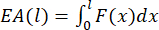(1)

where l is the crushing distance, and F(x) is the crushing force. To compare the energy absorption performance of each structure that was investigated in this study, the crushing distance was set to 20 mm.

The SEA16 indicates the energy absorbed per unit mass of the structure: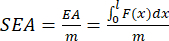(2)

where m is the mass of the structure.

The PCF is the peak crushing force during the whole compression process. The MCF38 is calculated as follows: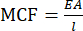(3)

The CFE4 is defined as the ratio of MCF to PCF: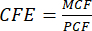(4)

The results of the quasi-static compression of the three thin-walled tubes were collected (step 2.9) from the simulations conducted in this study, and several main conclusions were obtained as follows.

The thickness gradient (step 2.2.2.2) changed the deformation mode of the ST and significantly improved the energy absorption performance. When the thickness variation factor k was 0.9, 1.2, or 1.5, the deformation mode of the ST changed from transverse expansion and contraction to axial progressive folding (Figure 4). The PCF decreased significantly, and the amplitude of force fluctuation became small (Figure 7). The EA and SEA increased significantly, and the CFE increased with increasing k (Figure 9). As calculated from Table 2, the SEA, EA, and CFE of the ST with k = 1.5 increased by 52.4%, 53.6%, and 199.7%, respectively, compared with the model with k = 0, while the PCF decreased by 48.6%. This indicates that the proposed single corrugated conical tube with variable thickness is an efficient energy absorber.

The introduction of lateral corrugation into the conical tube can improve the energy absorption performance of the tube. As shown in Table 2, the SEA and EA of both the ST and DT were higher than those of the CT. From the calculation in Table 2, the EA and SEA of the DT with k = 0 improved by 373% and 95.7%, respectively, compared with the CT with k = 0. Although the strengthening of the corrugated tube wall led to a significant increase in the PCF of the DT, the CFE of the DT was still 31.6% higher than that of the CT. Comparing the energy absorption evaluation indicators showed that introducing lateral corrugations is an effective method to improve the energy absorption capacity.

With an increase in k (step 2.2.2.2), the deformation mode of the CT tube changed from diamond-shaped progressive folding to circular-shaped progressive folding (Figure 5), while the DT tube always maintained a transverse expansion and contraction mode (Figure 6).

The effect of the thickness gradient on the PCF and CFE was significant. With k = 1.5 for the CT, ST, and DT, the PCF decreased by 66.0%, 48.6%, and 33.1%, respectively, compared with the model with k = 0, while the CFE increased by 199.5%, 199.7 and 63.9%, respectively. With higher k values, the buckling occurred closer to the loading end, where the cross-sectional thickness of the plastic fold is smaller. Therefore, the PCF also decreased (Figure 8). At the same time, the EA of the thin-walled tubes slightly changed with increasing k values, which also led to an increase in the CFE (Figure 10 and Figure 11).

To verify the finite elementmodel, a quasi-static compression experiment was conducted on a 3D-printed stainless steel CT with k = 0 (Figure 12A) using a computer-controlled universal testing machine (see Table of Materials).The force-displacement curves from the experiment and the simulation matched well, and the deformation patterns were almost identical (Figure 12B).The energy absorption evaluationindicators, which were obtained from the force-displacement curve through Equations 1-4, were also compared (Table 3). The errors associated with the PCF, MCF, and EA were 0.37%, 4.98%, and 4.98%, respectively, indicating that the finite element model can be considered effective.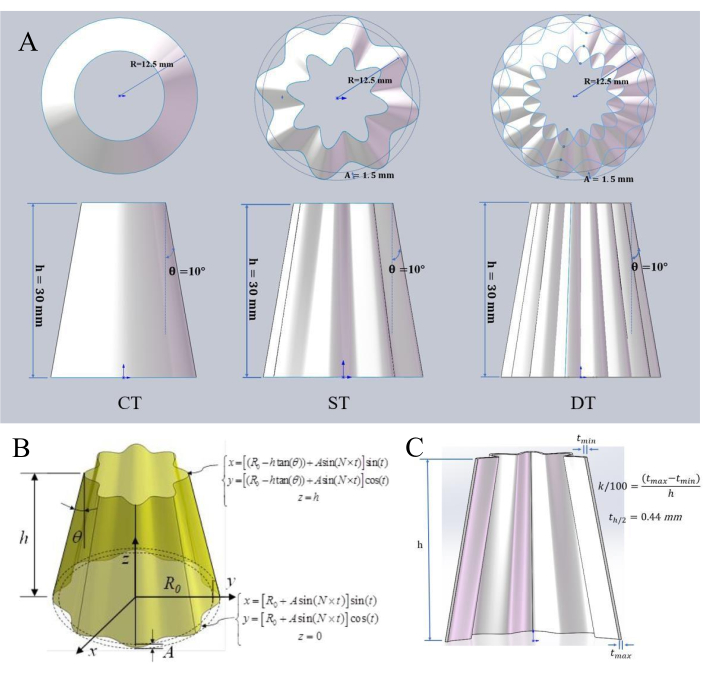Figure 1: Schematic diagram of three thickness gradient structures: CT, ST, and DT. (A) Schematic diagram of the conical tube (CT), single corrugated tapered tube (ST), and double corrugated tapered tube (DT). The height (h), base radius (R), and inclination angle (θ) of the three thin-walled tubes are 30 mm, 12.5 mm, and 10°, respectively. The corrugation amplitude (A) of the ST is 1.5 mm, the number of corrugations (N) is eight, and the DT is built by crossing two STs. (B) Geometric parameters: The cross-section of the ST is formed by a sine function. (C) Thickness gradient design. The upper end of the tube is thin, the lower end is thick, the gradient of the thickness is controlled by the thickness variation coefficient k, and k is set to 0, 0.3, 0.6, 0.9, 1.2, and 1.5. In addition, the thickness of half of the height is set to a fixed value (i.e., th/2  = 0.44 mm). Please click here to view a larger version of this figure.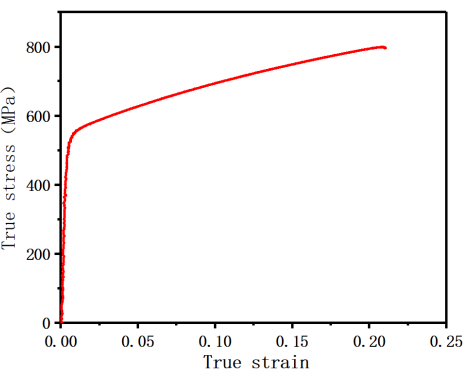Figure 2: True stress-true strain curve for 316 L stainless steel. The parameters, such as the Young's modulus, yield stress, and plastic strain, were obtained from the true stress-strain curve. Please click here to view a larger version of this figure.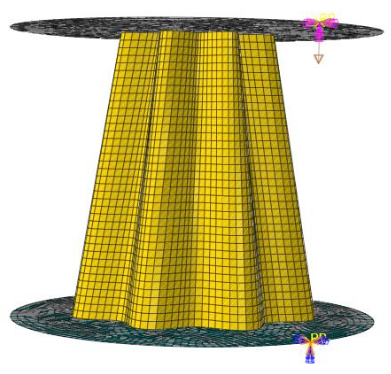Figure 3: Finite element model of the ST.The finite element model was generated after performing the Mesh step. Please click here to view a larger version of this figure.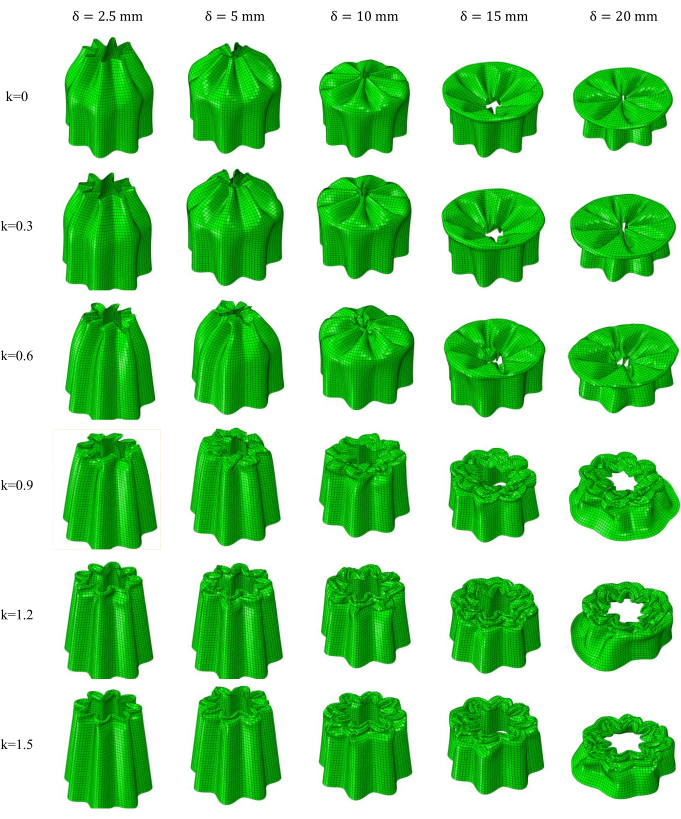Figure 4: Deformation modes of the ST with different k values. The deformation of the tube is obtained from the Visualization (step 2.8.3). δ denotes the compression displacement. Please click here to view a larger version of this figure.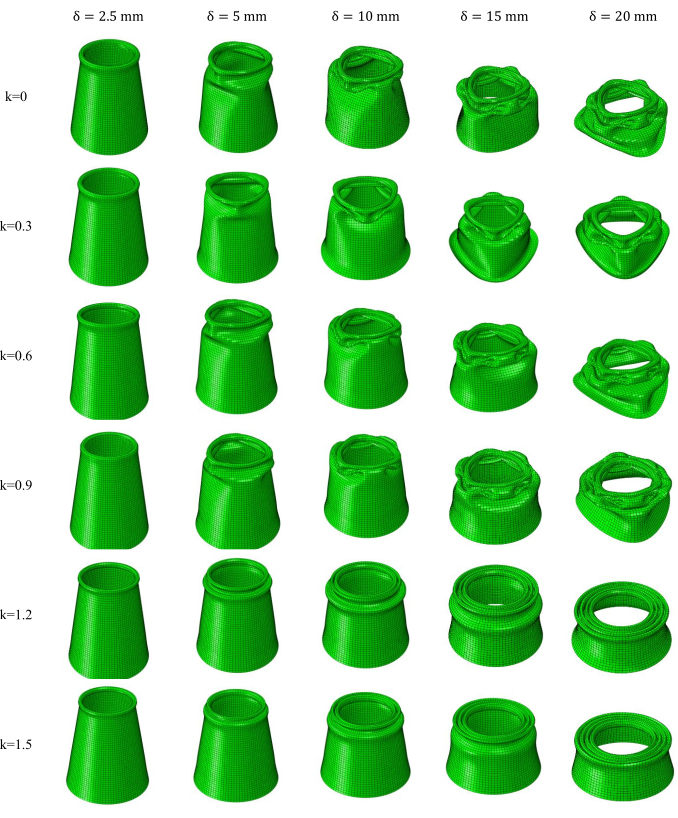Figure 5: Deformation modes of the CT with different k values. The deformation of the tube is obtained from the Visualization (step 2.8.3). Please click here to view a larger version of this figure.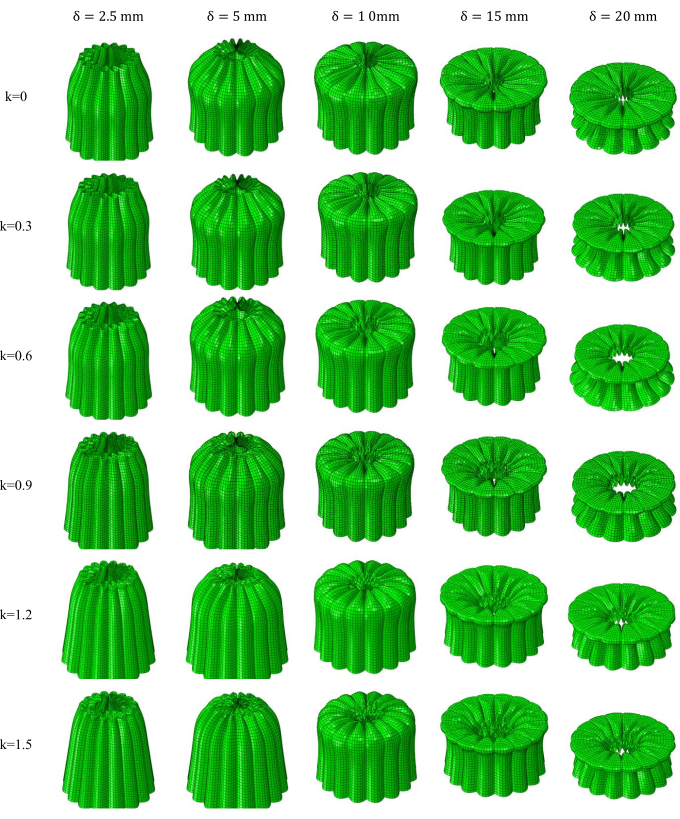Figure 6: Deformation modes of the DT with different k values. The deformation of the tube is obtained from the Visualization (step 2.8.3). Please click here to view a larger version of this figure.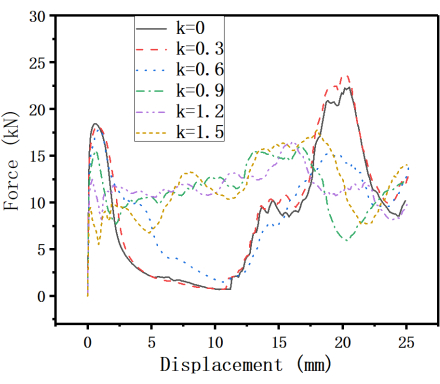Figure 7: Force-displacement curves of the ST with different k values. The force-displacement curve of the ST changes greatly with increasing k. Please click here to view a larger version of this figure.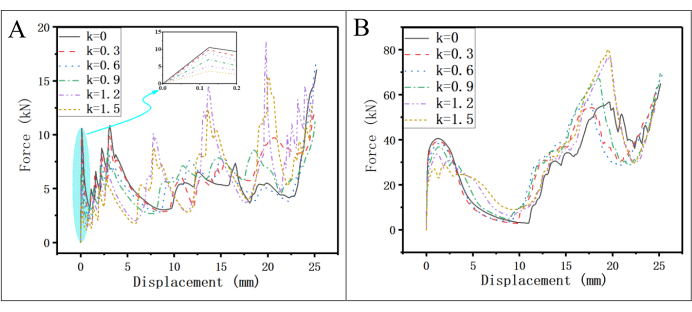Figure 8: Force-displacement curves of the CT and DT with different k values. (A) CT; (B) DT. The force-displacement curves are exported in the Job step. Please click here to view a larger version of this figure.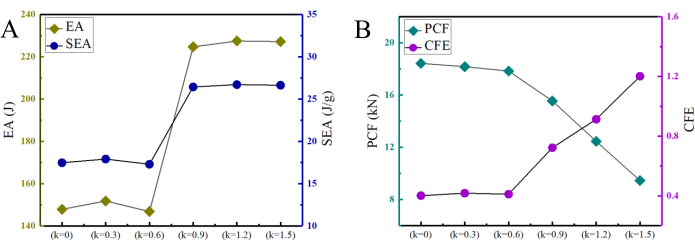Figure 9: Energy absorption performance of the ST with different k values. (A) Total energy absorption (EA) and specific energy absorption (SEA); (B) Peak crushing force (PCF) and crushing force efficiency (CFE). The energy absorption performance is calculated using Equations 1-4. Please click here to view a larger version of this figure.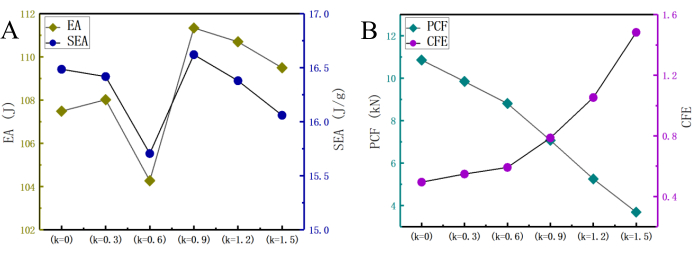Figure 10: Energy absorption performance of the CT with different k values. (A) EA and SEA; (B) PCF and CFE. The energy absorption performance is calculated using Equations 1-4. Please click here to view a larger version of this figure.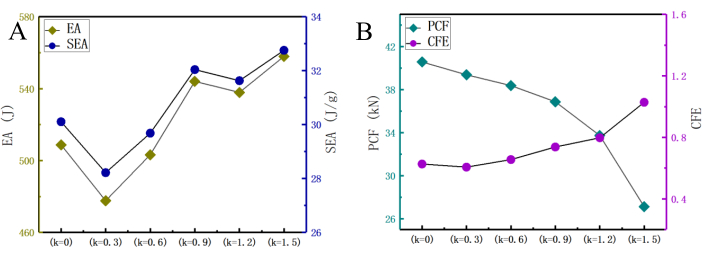Figure 11: Energy absorption performance of the DT with different k values. (A) EA and SEA; (B) PCF and CFE. The energy absorption performance is calculated using Equations 1-4. Please click here to view a larger version of this figure.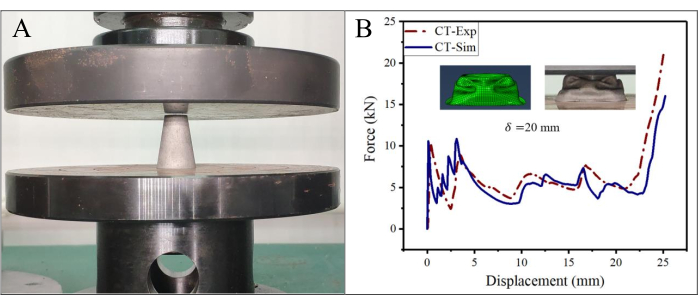Figure 12: Comparison between the experimental and finite element simulations. (A) Quasi-static compression test of the CT; (B) force-displacement curves and deformation patterns of the CT. (A) The quasi-static axial compression test was carried out using a computer-controlled universal testing machine. The CT was placed between the upper and lower platens. During the test, the lower platen was fixed, and the upper platen was moved downward at a speed of 3 mm/min. The force and displacement data were obtained by the sensor of the upper platen. Please click here to view a larger version of this figure.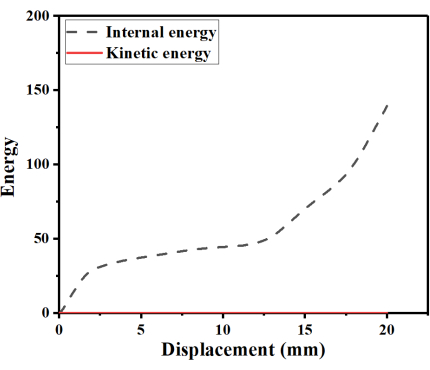Figure 13: Energy-displacement curves. The energy-displacement curves are exported in the Job step. Please click here to view a larger version of this figure.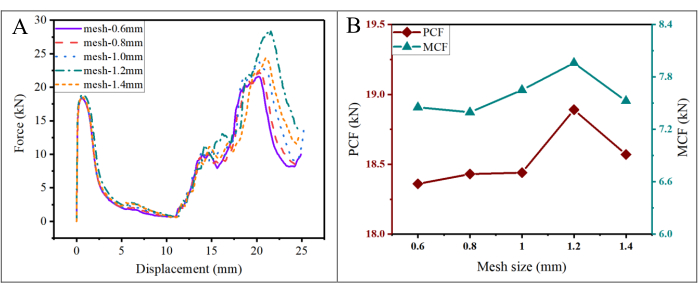Figure 14: Mesh convergence analysis of the ST (k = 0). (A) Force-displacement curves; (B) PCF and MCF. In the mesh step, the mesh size is set to 0.6 mm, 0.8 mm, 1.0 mm, 1.2 mm, and 1.4 mm. Then, the force-displacement curves for the models with the five mesh sizes are derived from the Job step. Please click here to view a larger version of this figure.

Table 1: Sketch parameters of the CT, ST, and DT. Cross-sectional shapes and parameters of the upper and lower ends of three types of thin-walled tubes. Please click here to download this Table.

Table 2: Crashworthiness evaluation index of the thin-walled tubes with different k values. The energy absorption performance is calculated using Equations 1-4. Please click here to download this Table.

### Discussion

The quasi-static compression performance of tapered tubes was studied by finite element analysis. Two new types of corrugated tapered tubes with variable thicknesses were designed, and their quasi-static compression performance was investigated. In quasi-static compression simulations, some important steps and settings need to be verified.

The material parameters are the basic requirements for the finite element calculation (step 2.2.1 of the protocol). In this study, the material parameters were obtained from the literature41. Standard tensile tests on 316 L stainless steel were conducted, and the nominal stress-strain curve of the material was obtained. Then, the nominal stress-strain curve was transformed into the true stress-strain curve (Figure 2) using Equation 5 and Equation 6. Finally, parameters such as the Young's modulus, yield stress, and plastic strain were obtained from the true stress-strain curve.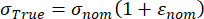(5)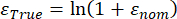(6)

The quasi-static compression simulation should be set with a reasonable loading speed. The loading speed range of standard quasi-static compression experiments is generally between 0.01-1 mm/s44. However, such a loading speed is too slow for numerical simulations. This is because the explicit time integration method is only conditionally stable for very small time increments. To reduce the calculation time, for a quasi-static analysis, the velocity scaling method is often used to speed up the crushing velocity44. Therefore, the same method was also used in this study, where a loading speed of 0.5 m/s (step 2.6.2) was set for the top plane. Theoretically, when the ratio of total kinetic energy to total internal energy is less than 5%, it is assumed that a quasi-static state has been maintained8. The numerical results showed that the kinetic energy was much smaller than the internal energy and that the ratio of the kinetic to internal energy was less than 1% (Figure 13). This means that the inertia effect is minor and that the dynamic effect can be ignored in the simulation.

The mesh size also needs to be verified (step 2.7.1). A mesh that is too large will lead to non-convergence of the computational results, while a mesh that is too small will lead to long computation times. Therefore, a reasonable mesh size needs to be selected. In this study, the simulation results were compared for mesh sizes of 0.6 mm, 0.8 mm, 1.0 mm, 1.2 mm, and 1.4 mm, as shown in Figure 14. The force-displacement curves with mesh sizes of 0.6 mm and 0.8 mm were very close. In addition, the PCF and MCF tended to converge when the mesh size was less than 0.8 mm. When the mesh size was reduced from 0.8 mm to 0.6 mm, the MCF increased from 7.396 kN to 7.453 kN, while the PCF decreased from 18.43 kN to 18.36 kN. Both changes are within 1%, which is acceptable. Therefore, considering the computational efficiency, the final mesh size was determined to be 0.8 mm.

Finite element simulation plays an important role in the initial feasibility studies of thin-walled structures.In addition, the finite element method can obtain data that are difficult to obtain via experimental methods. For example, the structural deformation of various sections and angles of view, the stress at any position, and the values of various energies and their proportions45 are very helpful for analyzing the problem. Thus, finite element simulation can be used as an alternative to experiments to save time and resources. The results obtained from the finite element simulation in this study showed good agreement with the results of a representative quasi-static compression experiment (Figure 12).

Despite its advantages, the simulation method employed in this study is limited to quasi-static testing and is not applicable to other working conditions, such as a bullet impact or a hammer impact. For dynamic loading, the strain rate sensitivity and damage parameters of the material should be included (in the property step 2.2 in the protocol)46. In addition, in some complex structures, it is difficult to mesh the models, resulting in large deviations in the calculation results.

In the future, this method will be widely used to study the crashworthiness of energy-absorbing structures. Researchers are committed to designing and manufacturing thin-walled structures with better energy absorption performance43, and the finite element method is the most direct, effective, and economical method to help judge structural performance.In addition, with the improvement of computing speed and software updates, with the finite element method will be able to establish the analysis model more accurately and quickly and, thus, obtain more accurate results. This will enable the application of the finite element method to more fields,such as aerospace, transportation, and construction engineering.

### Disclosures

The authors have nothing to disclose.

### Acknowledgments

The first author would like to acknowledge grants from the National Natural Science Foundation of China (No. 52078152 and No. 12002095), General Program of Guangzhou Science and Technology Plan (No. 202102021113), Guangzhou Government-University Union Fund (No. 202201020532), and Guangzhou Municipal Science and Technology Project (Grant No. 202102020606).

### Materials

 Name Company Catalog Number Comments ABAQUS Dassault SIMULIA Finite element software CT Botong 3D printing Conical tube for experiment SOLIDWORKS Dassault Systemes CAD software Universal testing machine SUNS UTM5205, 200kN

Video Coming Soon
DOIX